You’ve reached the end of your free Videos limit.
#11 | Graphs in Adiabatic Process: 1
(Physics) > Thermodynamics
Unable to watch the video, please try another server
Related Practice Questions :

In given process dW = 0, dQ < 0 then for a gas

1.  Temperature -  increases

2.  Volume - decreases

3.  Pressure - decreases

4.  Pressure - increases

High Yielding Test Series + Question Bank - NEET 2020

Difficulty Level:

The ratio of the relative rise in pressure for adiabatic compression to that for isothermal compression is

(1) $\gamma$

(2) $\frac{1}{\gamma }$

(3) 1-$\gamma$

(4) $\frac{1}{1-\gamma }$

High Yielding Test Series + Question Bank - NEET 2020

Difficulty Level:

The variation of pressure versus temperature of an ideal gas is shown in the given diagram. From this diagram, one can conclude that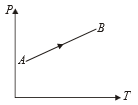1.  Volume increases continuously

2.  Volume decreases continuously

3.  Volume first increases then decreases

4.  Volume first decreases, then increase

High Yielding Test Series + Question Bank - NEET 2020

Difficulty Level:

The variation of density (p) of gas with its absolute temperature (T) at constant pressure is best represented by the graph

1.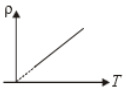2.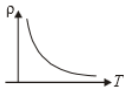3.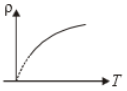4.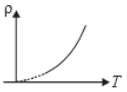High Yielding Test Series + Question Bank - NEET 2020

Difficulty Level:

An ideal gas with adiabatic exponent y is heated at constant pressure and it absorbs Q heat. What fraction of this heat is used to perform external work?

1.  $\mathrm{\gamma }$

2.  $\frac{\mathrm{\gamma }}{\mathrm{\gamma }}$

3.

4.